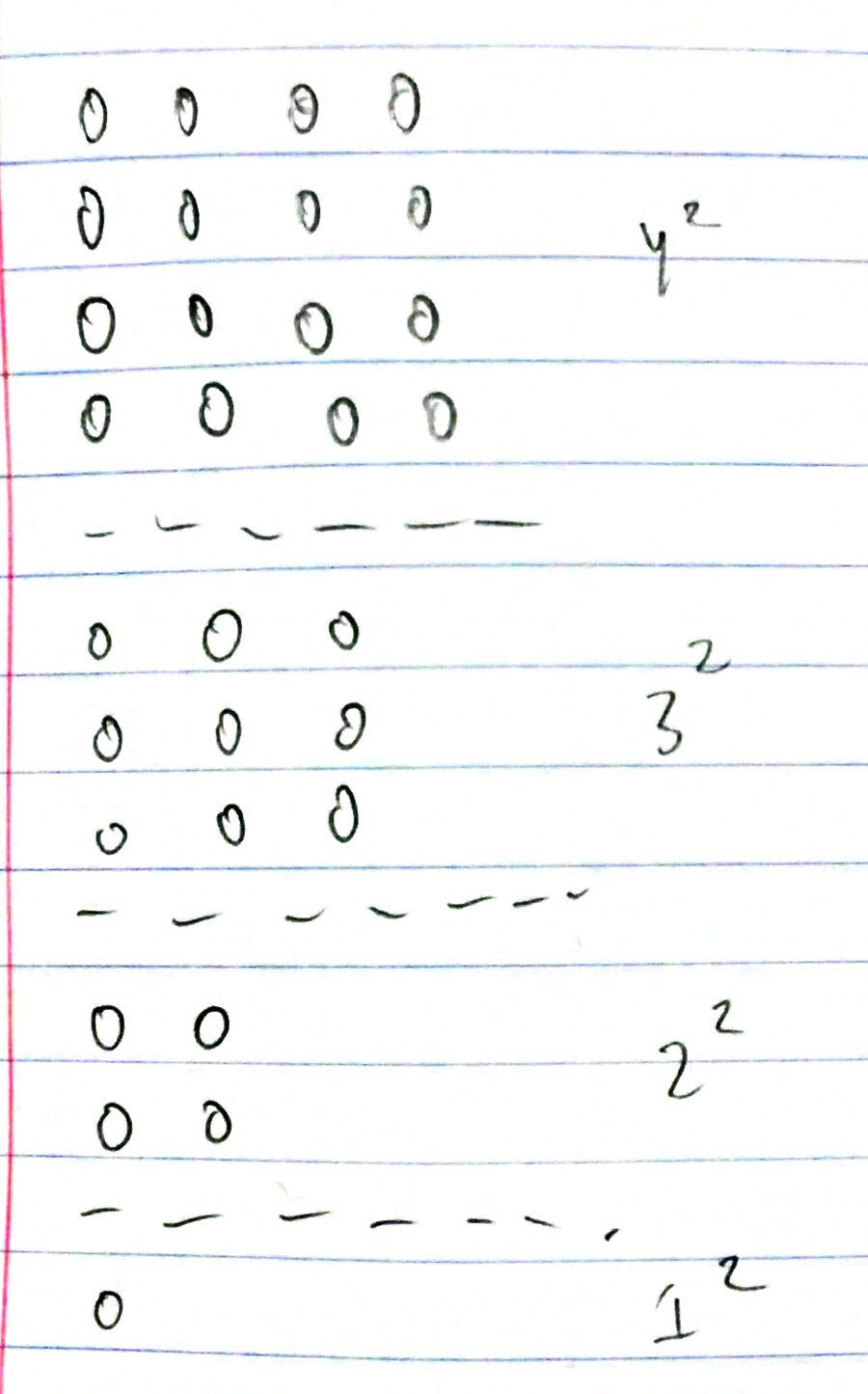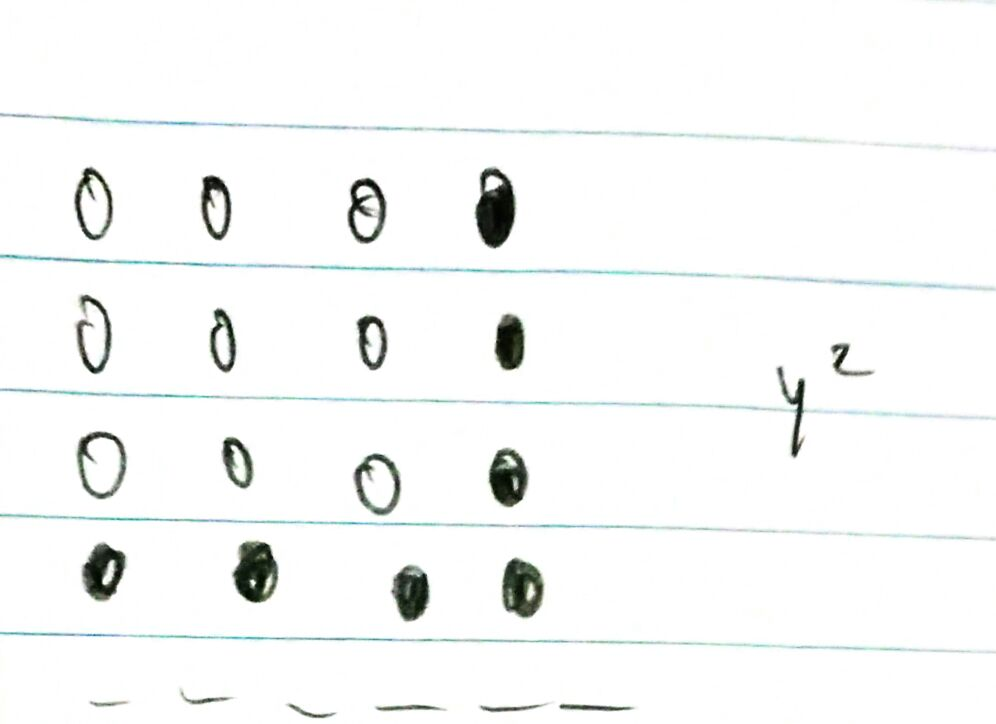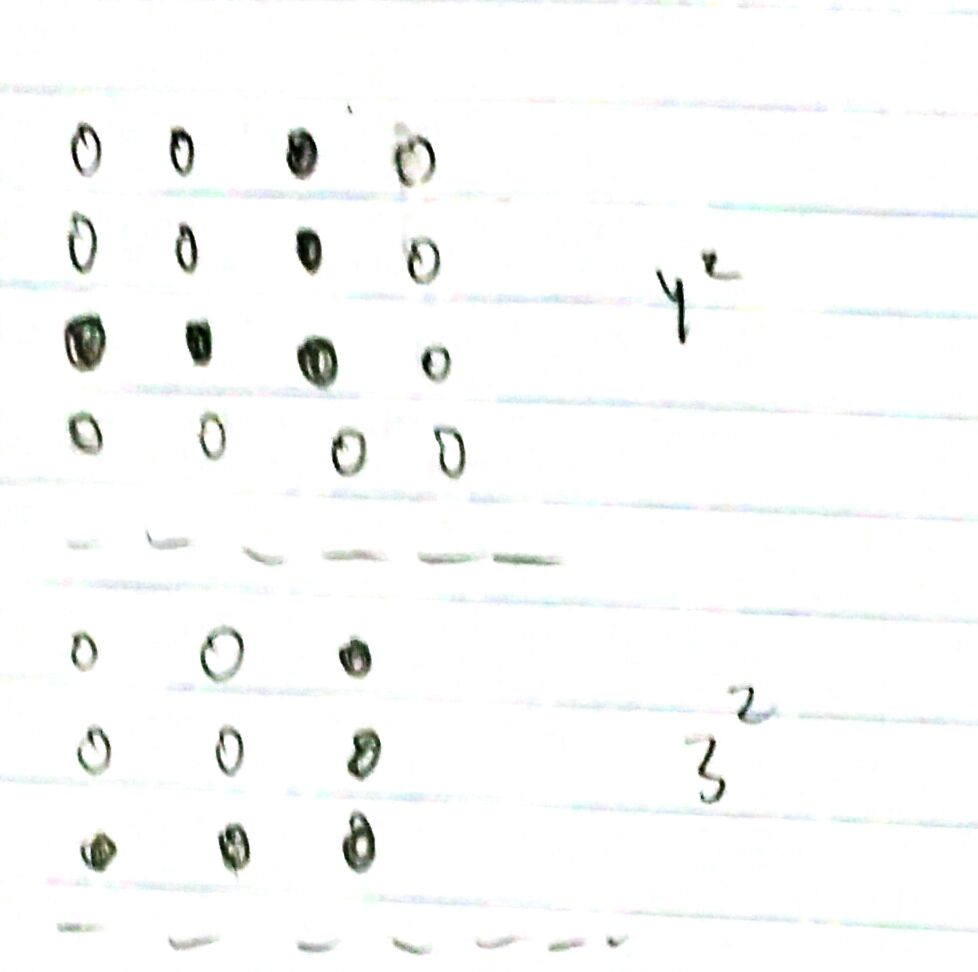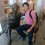# An Intuitive Geometric Proof of the Sum of n^2

Here's a proof of the identity $1^{2} + 2^{2} + 3^{2} + . . . + n^{2} = \frac{n(n+1)(2n+1)}{6}$

## Geometric Intuition

Think of it as stacking squares on top of each other to make a staircase/pyramid. Here's what the cross section looks like:Each layer is a horizontal cross section of that staircase. Our goal is to find a formula to sum up all of the balls.

In our case of $n = 4$, we can sum it up like this:

There are seven balls on the "first step" of our first layer. Here's what I mean:That's (1) times (7) = 7 balls

Now, for the "second step" in our staircase, we have to take into consideration the balls from the base layer and the layer above that.Now that's (2) times (5) = 10 balls

For the third step, we have to take into consideration the balls from the first, second and third layer.

That's (3) times (3)

The full sum looks like this: $(1)(7) + (2)(5) + (3)(3) + (4)(1)$

If you plug in 4 to the formula, it agrees with this sum, which is $\boxed{30}$.

## Now we see a pattern.

For the first term, it's $1 \cdot (2(4) - 1)$

For the second term, it's $2 \cdot (2(4) - 3)$

For the $i$th term, it's $(i)(2n - 2i+1)$

Play around with it and plug some numbers in, and you will see that this is the case.

This agrees with the theorem that the sum of consecutive odd numbers is a perfect square. Here's a diagram to refresh your memory:## Algebraic Manipulation

We have

$\displaystyle \sum_{k=1}^{n} k^2 = (1)(2n - 1) + (2)(2n - 3) + (3)(2n - 5) . . . (n)(1)$

which can be represented as

$\displaystyle \sum_{k=1}^{n} k^2 = \sum_{k=1}^{n} (k)(2n - 2k + 1)$

Algebra magic

$\displaystyle \sum_{k=1}^{n} (2nk - 2k^2 + k) = \displaystyle \sum_{k=1}^{n} k^2$

$3\displaystyle \sum_{k=1}^{n} k^2 = \displaystyle \sum_{k=1}^{n} (2nk + k)$

We know that $\boxed\sum_{k=1}^{n} = \frac{n(n+1)}{2}}$

$3\displaystyle \sum_{k=1}^{n} k^2 = \frac{2n \cdot n(n+1)}{2} + \frac{n(n+1)}{2}$

Therefore:

$\displaystyle \sum_{k=1}^{n} k^2 = \frac{(2n+1)n(n+1)}{6} = \frac{n(n+1)(2n+1)}{6}$

# QED :-)Note by Timothy Samson
3 years, 4 months ago

This discussion board is a place to discuss our Daily Challenges and the math and science related to those challenges. Explanations are more than just a solution — they should explain the steps and thinking strategies that you used to obtain the solution. Comments should further the discussion of math and science.

When posting on Brilliant:

• Use the emojis to react to an explanation, whether you're congratulating a job well done , or just really confused .
• Ask specific questions about the challenge or the steps in somebody's explanation. Well-posed questions can add a lot to the discussion, but posting "I don't understand!" doesn't help anyone.
• Try to contribute something new to the discussion, whether it is an extension, generalization or other idea related to the challenge.
• Stay on topic — we're all here to learn more about math and science, not to hear about your favorite get-rich-quick scheme or current world events.

MarkdownAppears as
*italics* or _italics_ italics
**bold** or __bold__ bold
- bulleted- list
• bulleted
• list
1. numbered2. list
1. numbered
2. list
Note: you must add a full line of space before and after lists for them to show up correctly
paragraph 1paragraph 2

paragraph 1

paragraph 2

[example link](https://brilliant.org)example link
> This is a quote
This is a quote
    # I indented these lines
# 4 spaces, and now they show
# up as a code block.

print "hello world"
# I indented these lines
# 4 spaces, and now they show
# up as a code block.

print "hello world"
MathAppears as
Remember to wrap math in $$ ... $$ or $ ... $ to ensure proper formatting.
2 \times 3 $2 \times 3$
2^{34} $2^{34}$
a_{i-1} $a_{i-1}$
\frac{2}{3} $\frac{2}{3}$
\sqrt{2} $\sqrt{2}$
\sum_{i=1}^3 $\sum_{i=1}^3$
\sin \theta $\sin \theta$
\boxed{123} $\boxed{123}$

## Comments

Sort by:

Top Newest

Nice proof

- 2 years, 9 months ago

Log in to reply

×

Problem Loading...

Note Loading...

Set Loading...